# Frank Solutions for Class 10 Maths Chapter 10 Remainder and Factor Theorems

Frank Solutions for Class 10 Maths Chapter 10 Remainder and Factor Theorems are provided here. These solutions are most valuable, that assist students in learning complex and challenging topics. The solutions serve as an important study tool when it comes to preparing for the exam. Hence, students are suggested to solve all the questions provided in the Frank Solutions for Class 10 Maths Chapter 10, to overcome mistakes while answering the questions.

Chapter 10 – Remainder and Factor Theorems are actually two theorems that relate the roots of a polynomial with its linear factors. The theorem is often used to help factorize polynomials without the use of long division. These Frank Solutions are solved in such a way that students can never get confused.

## Download the PDF of Frank Solutions for Class 10 Maths Chapter 10 Remainder and Factor Theorems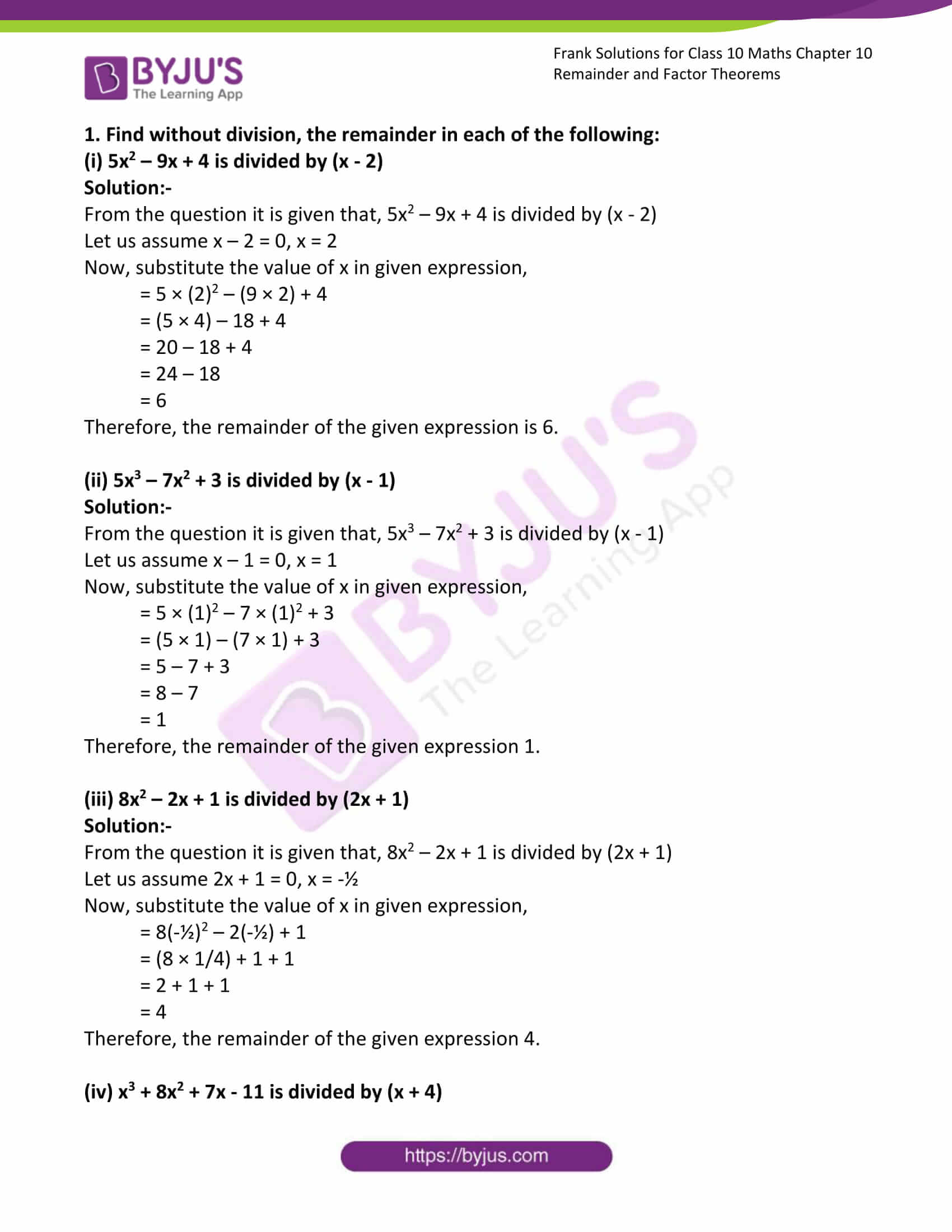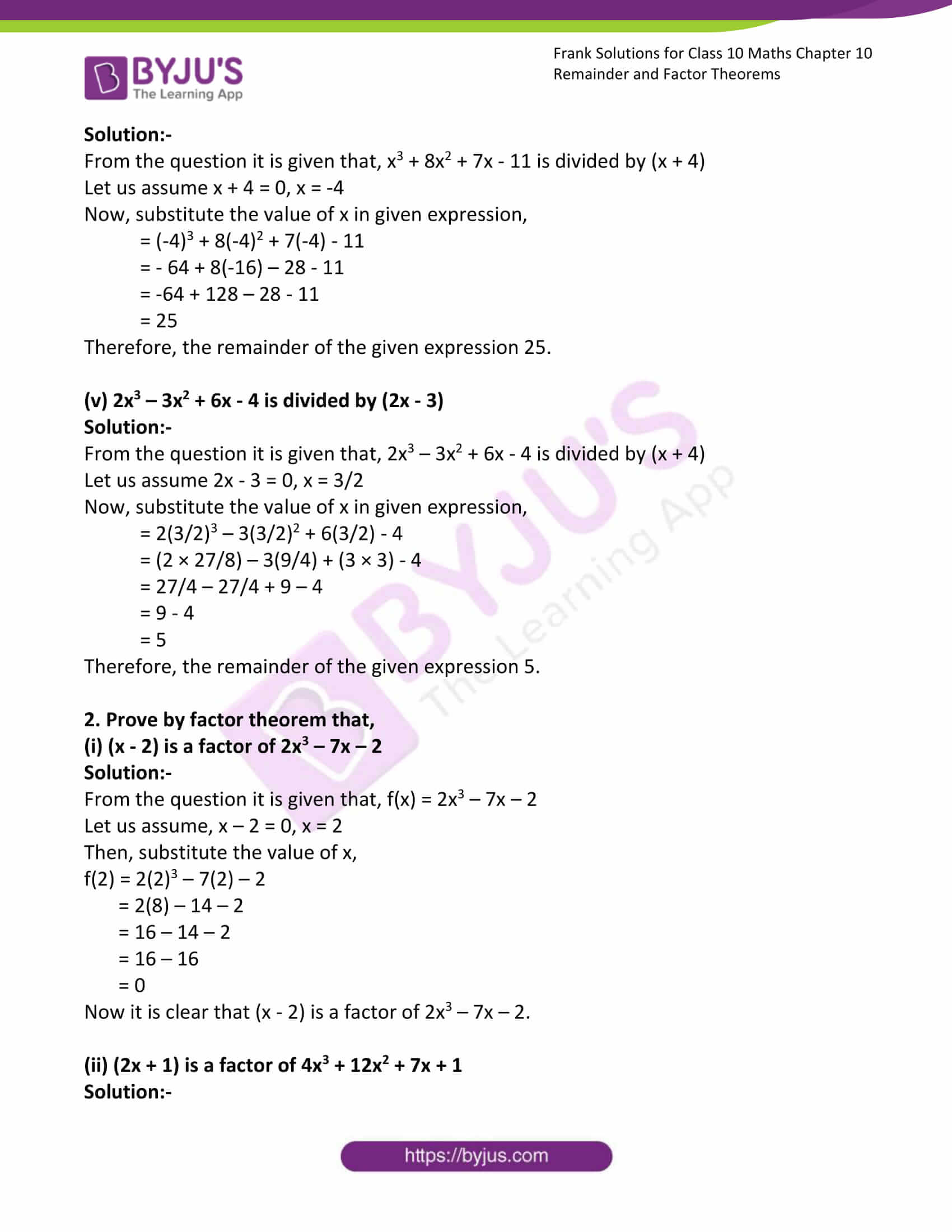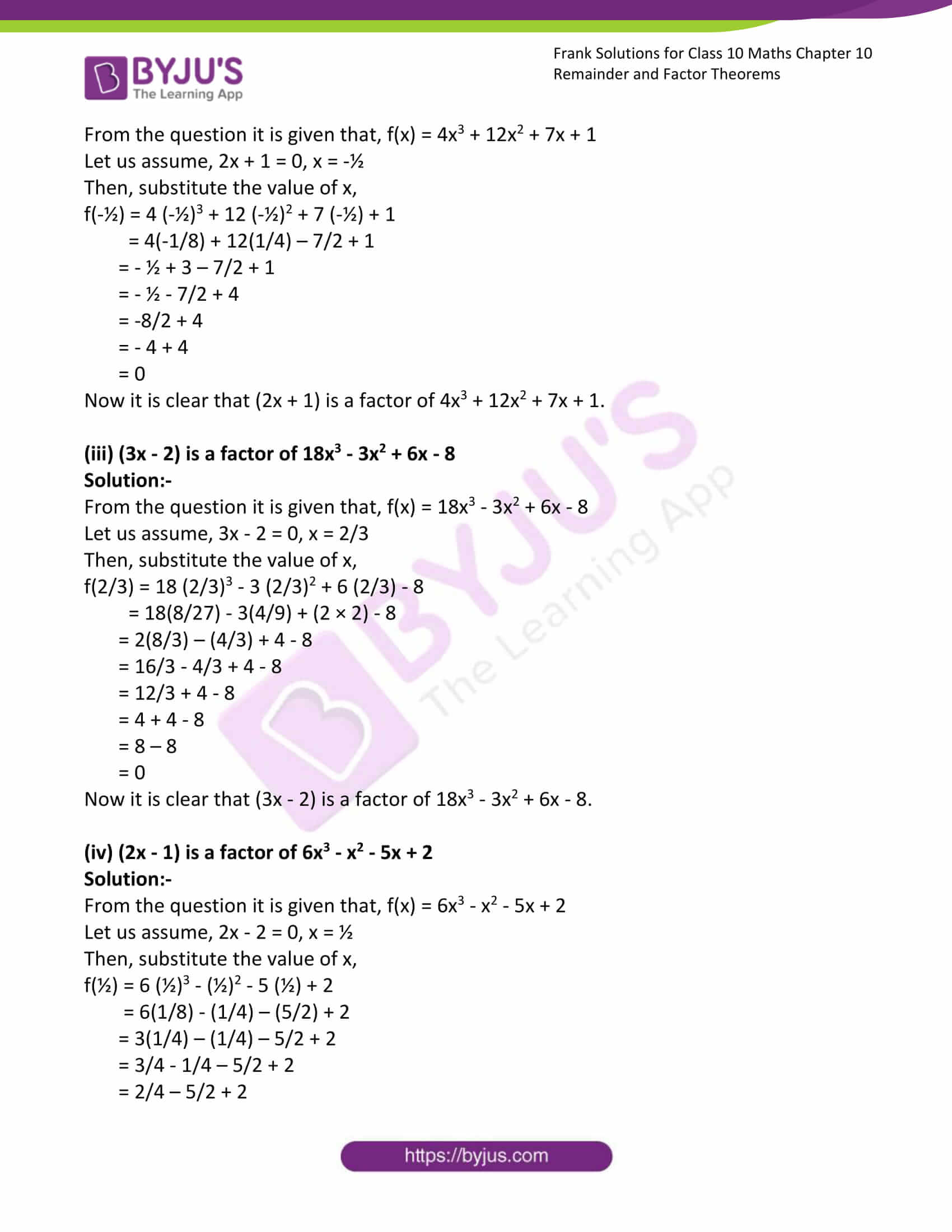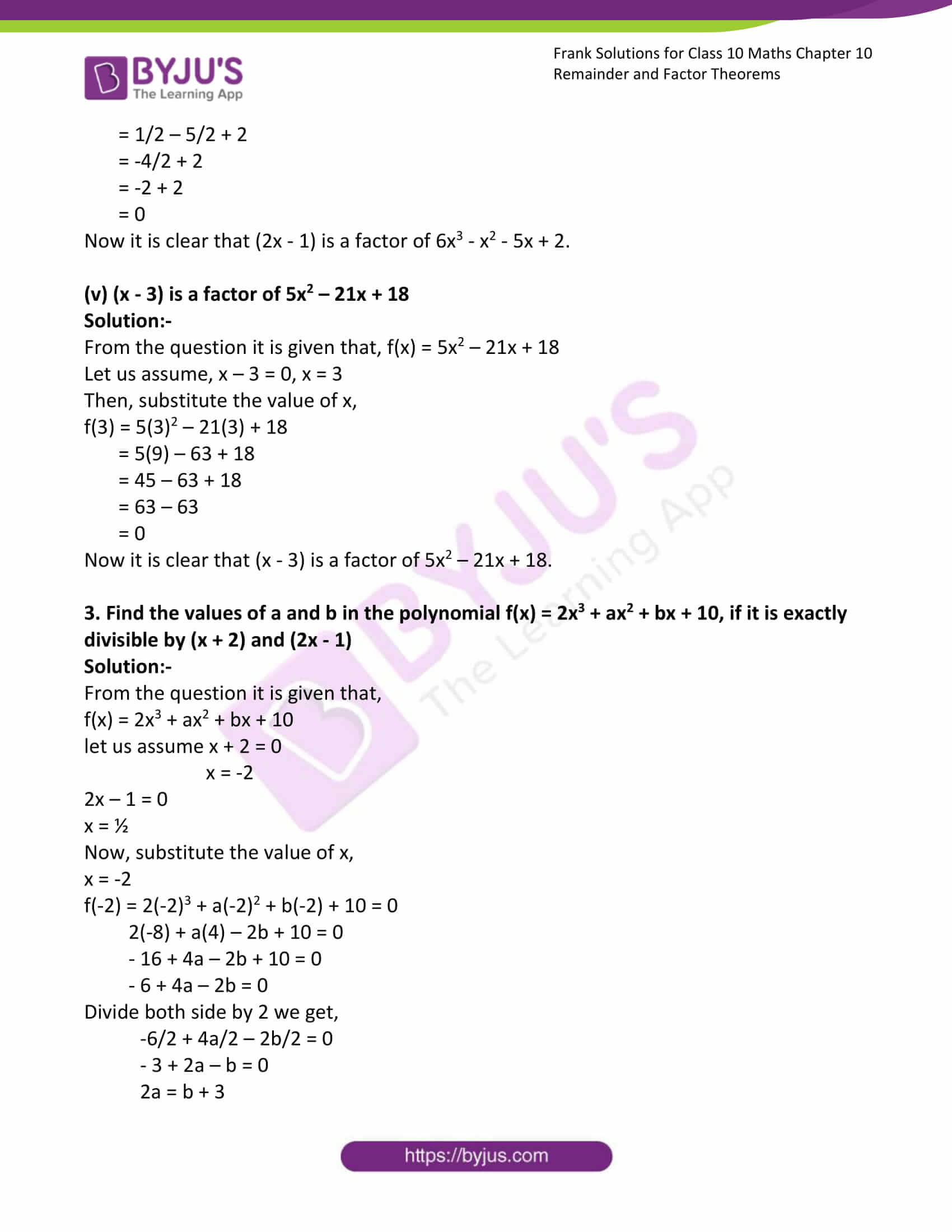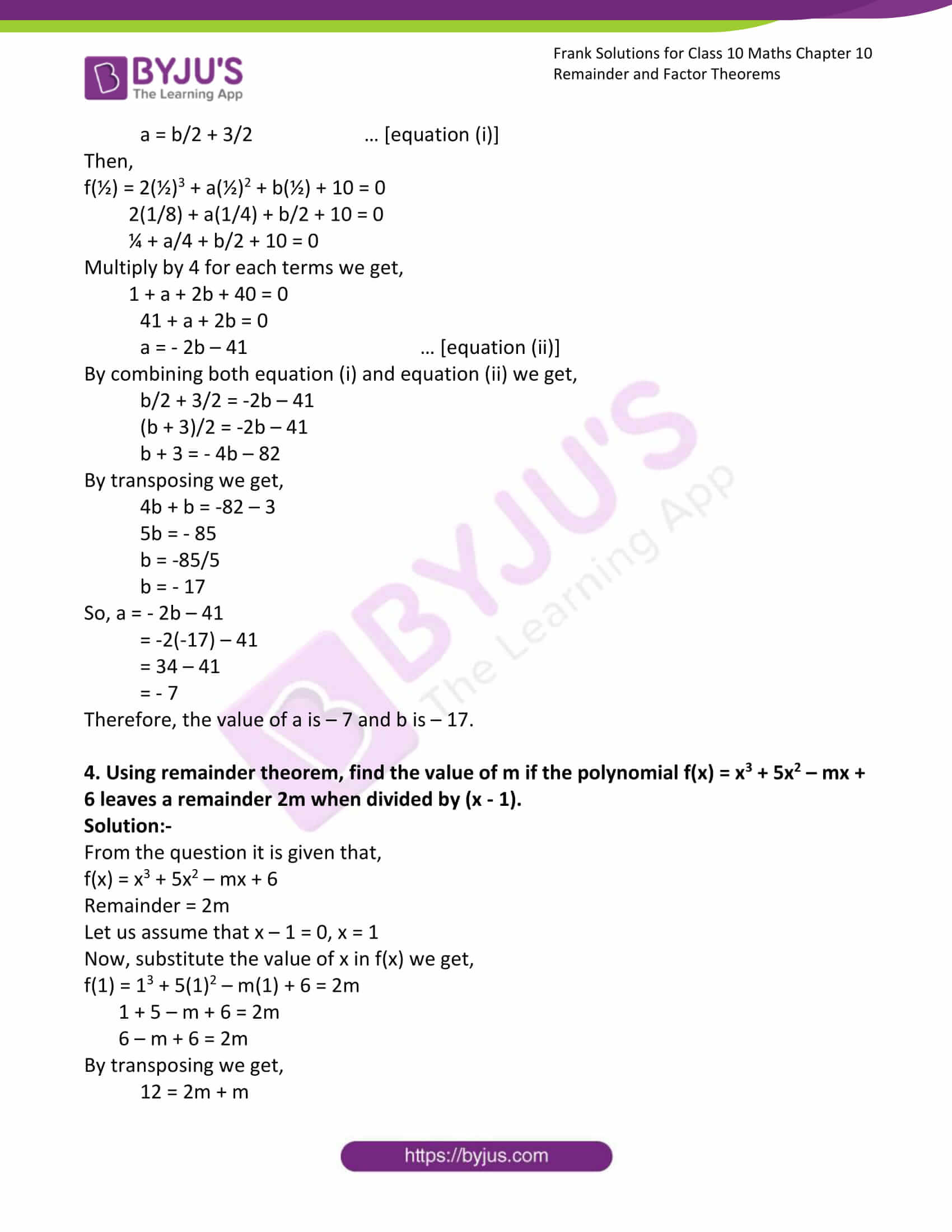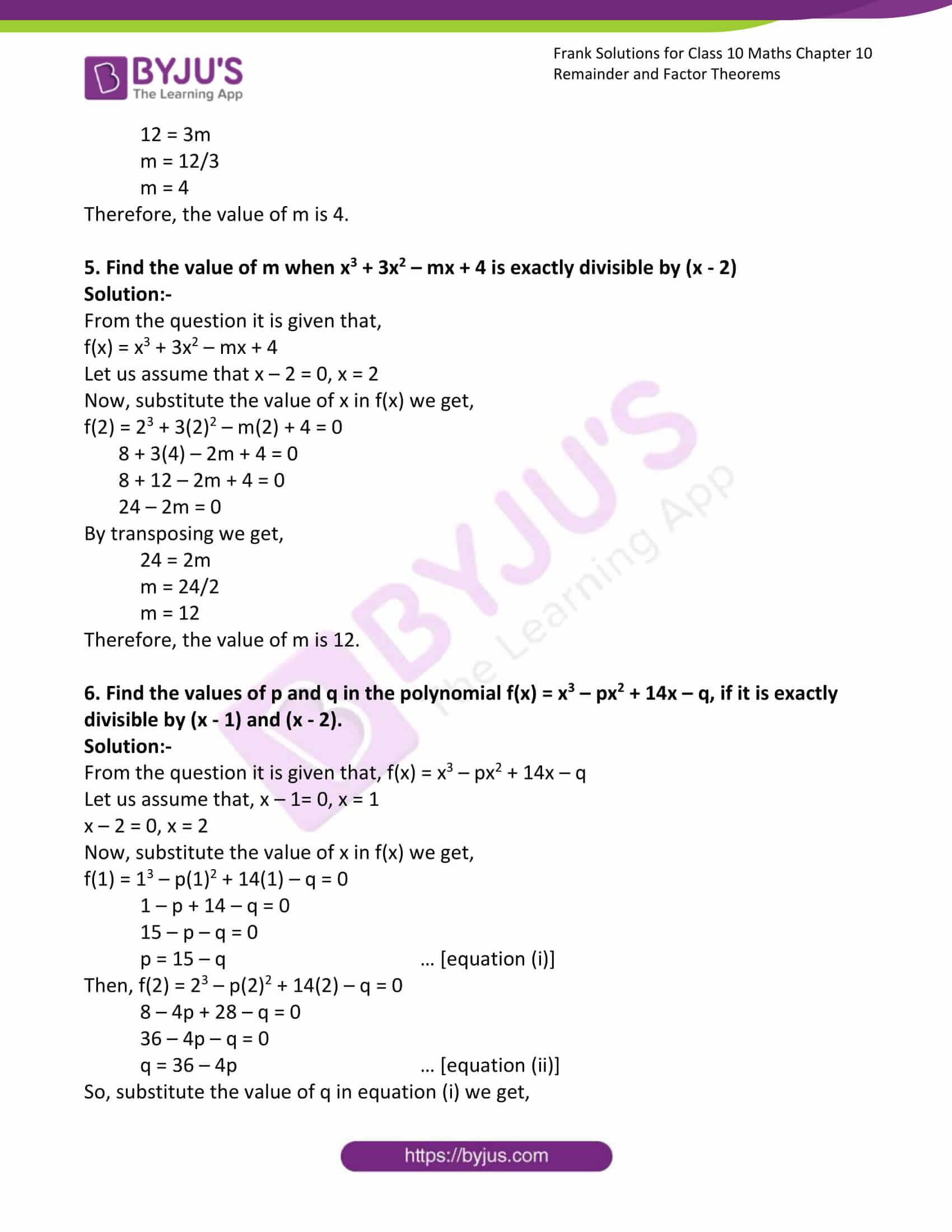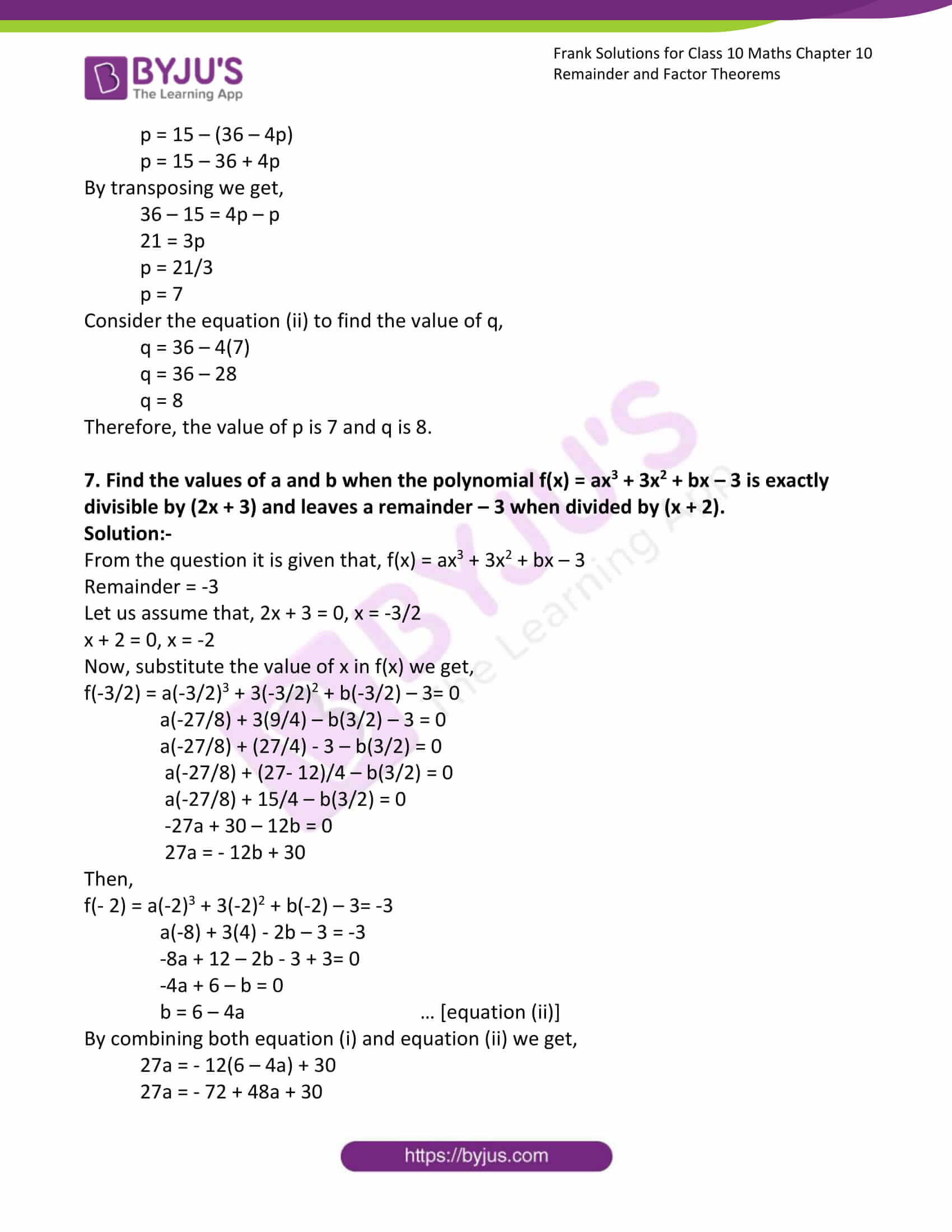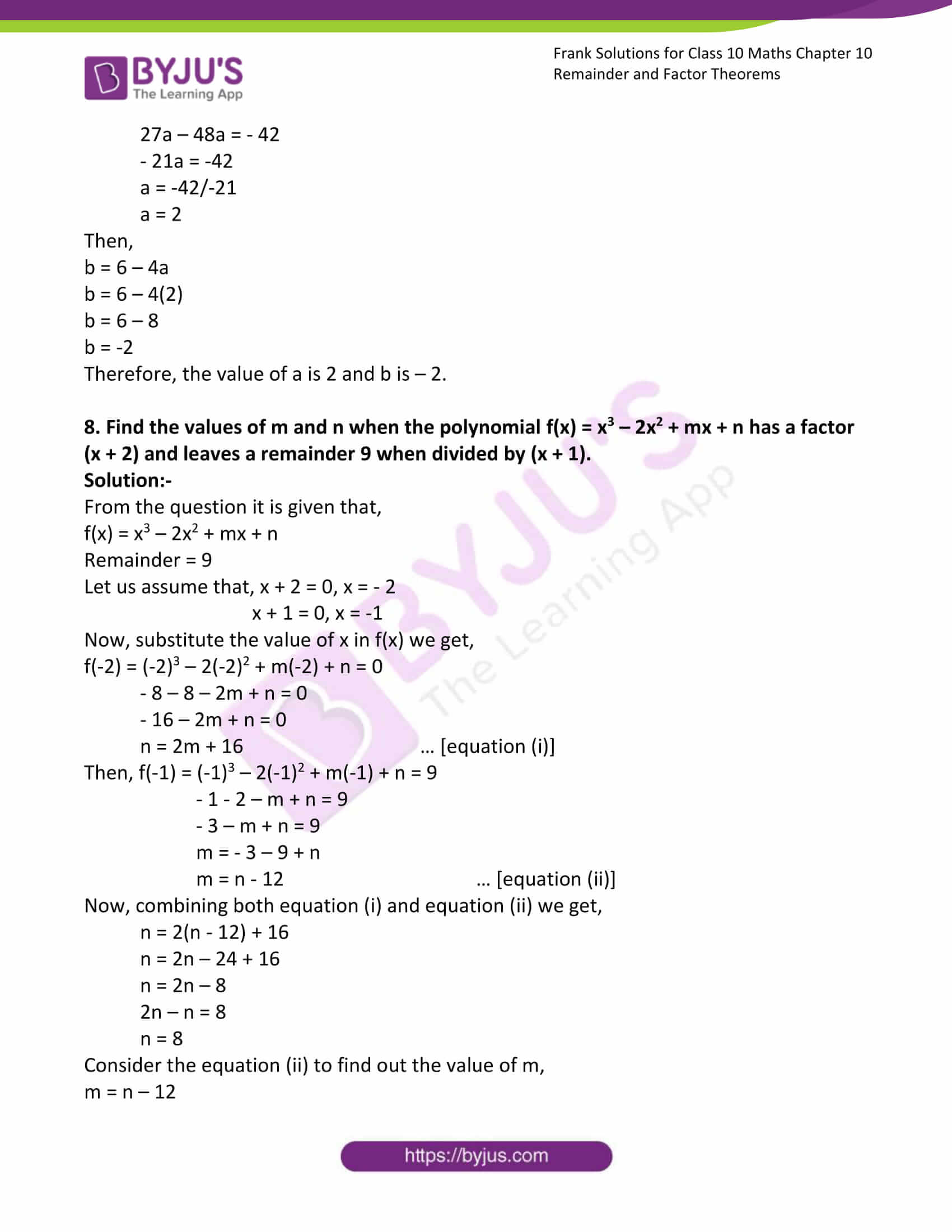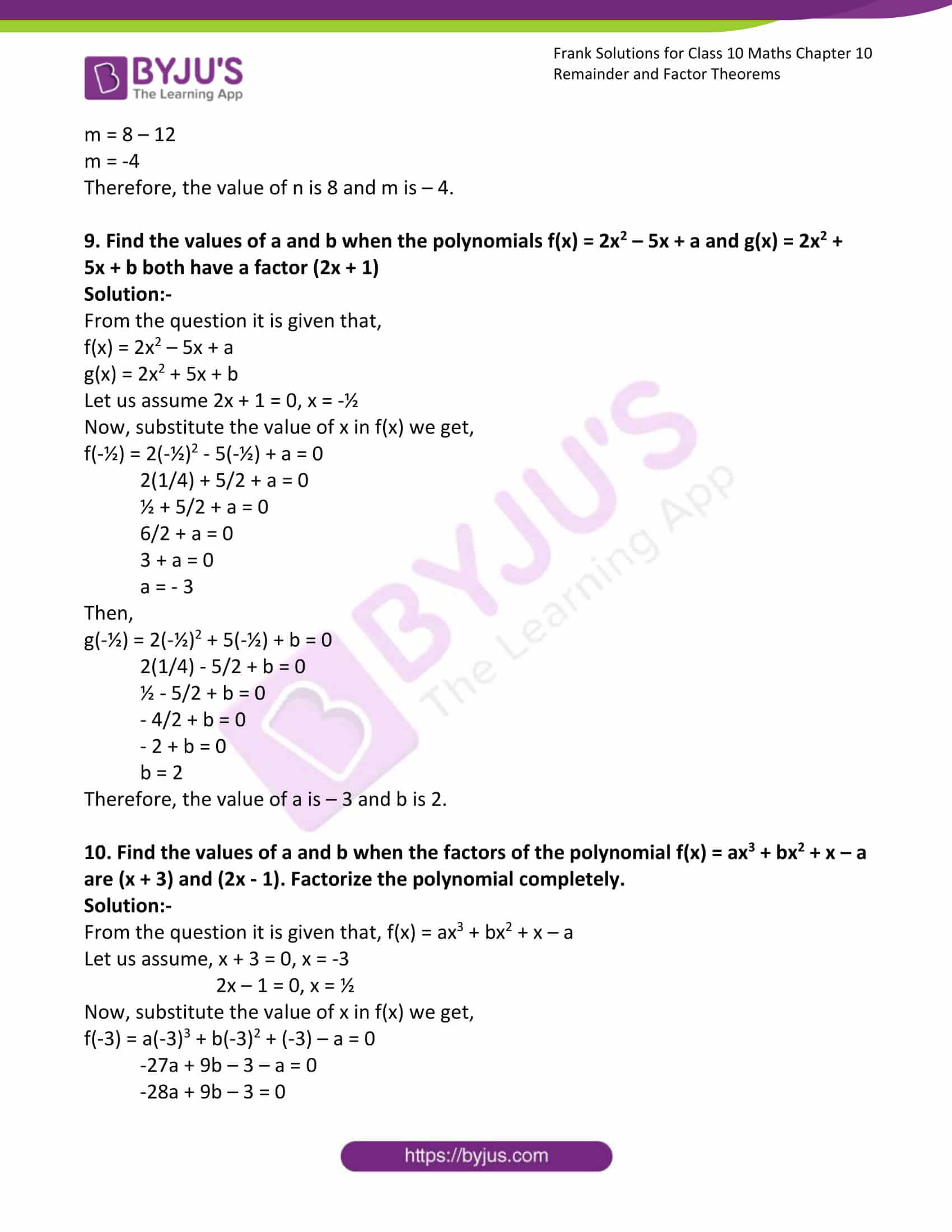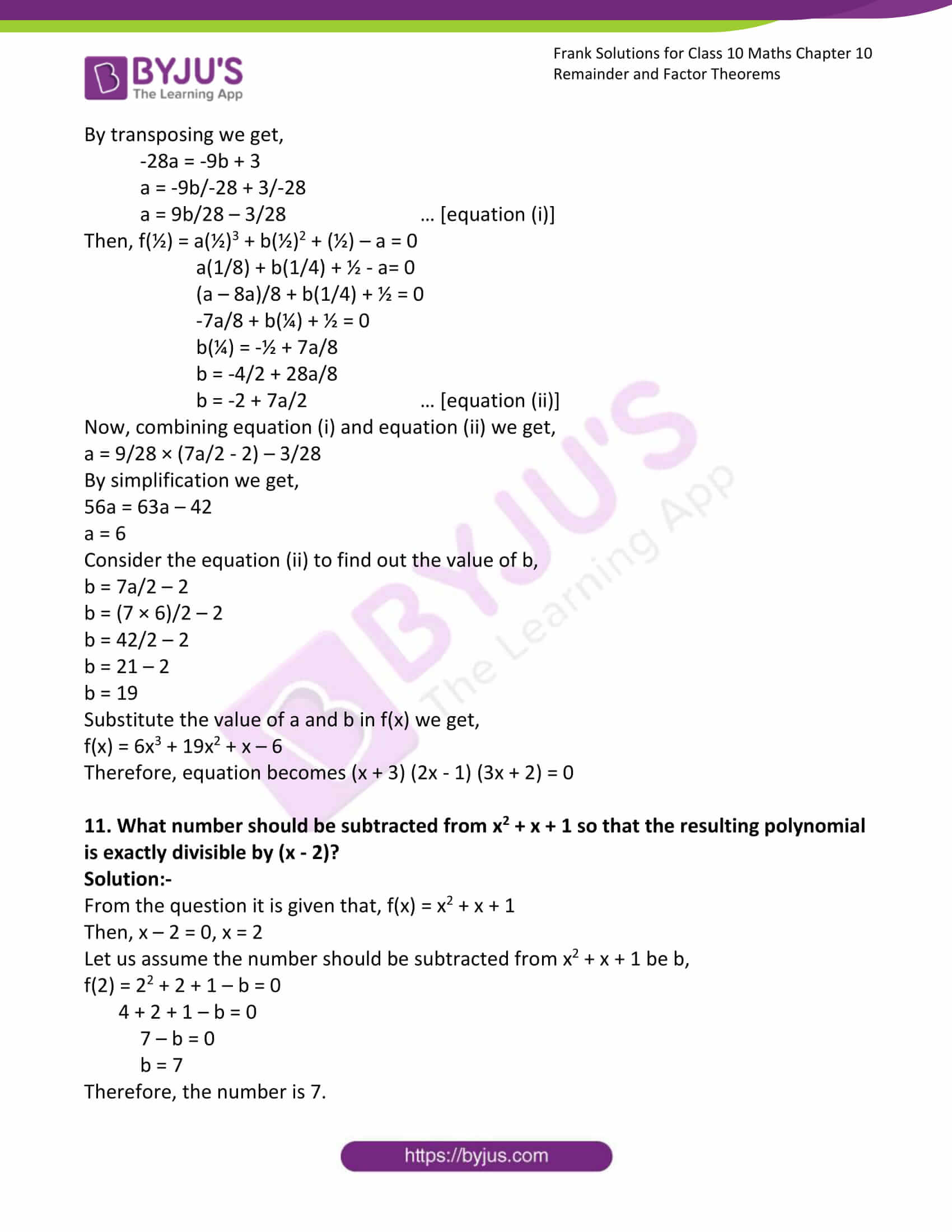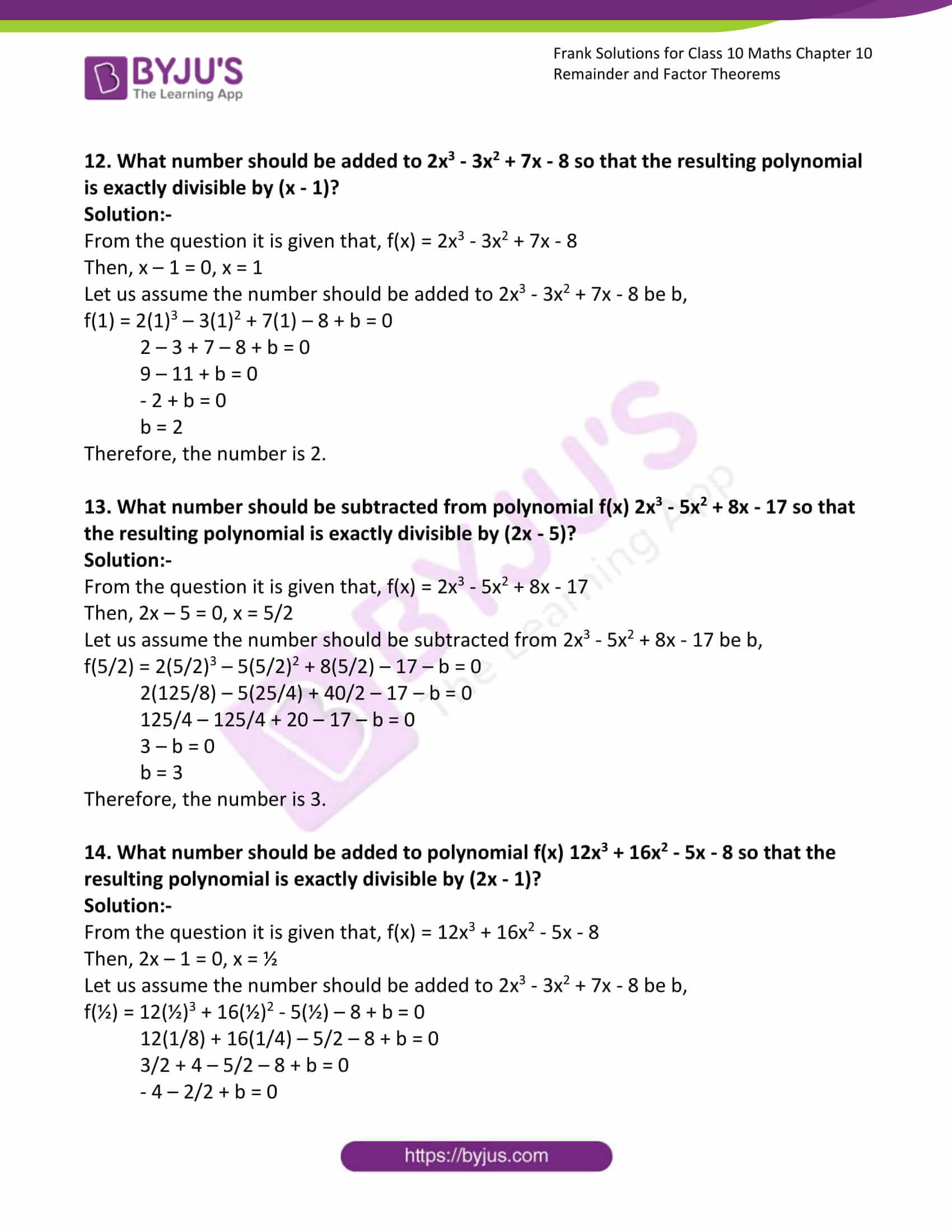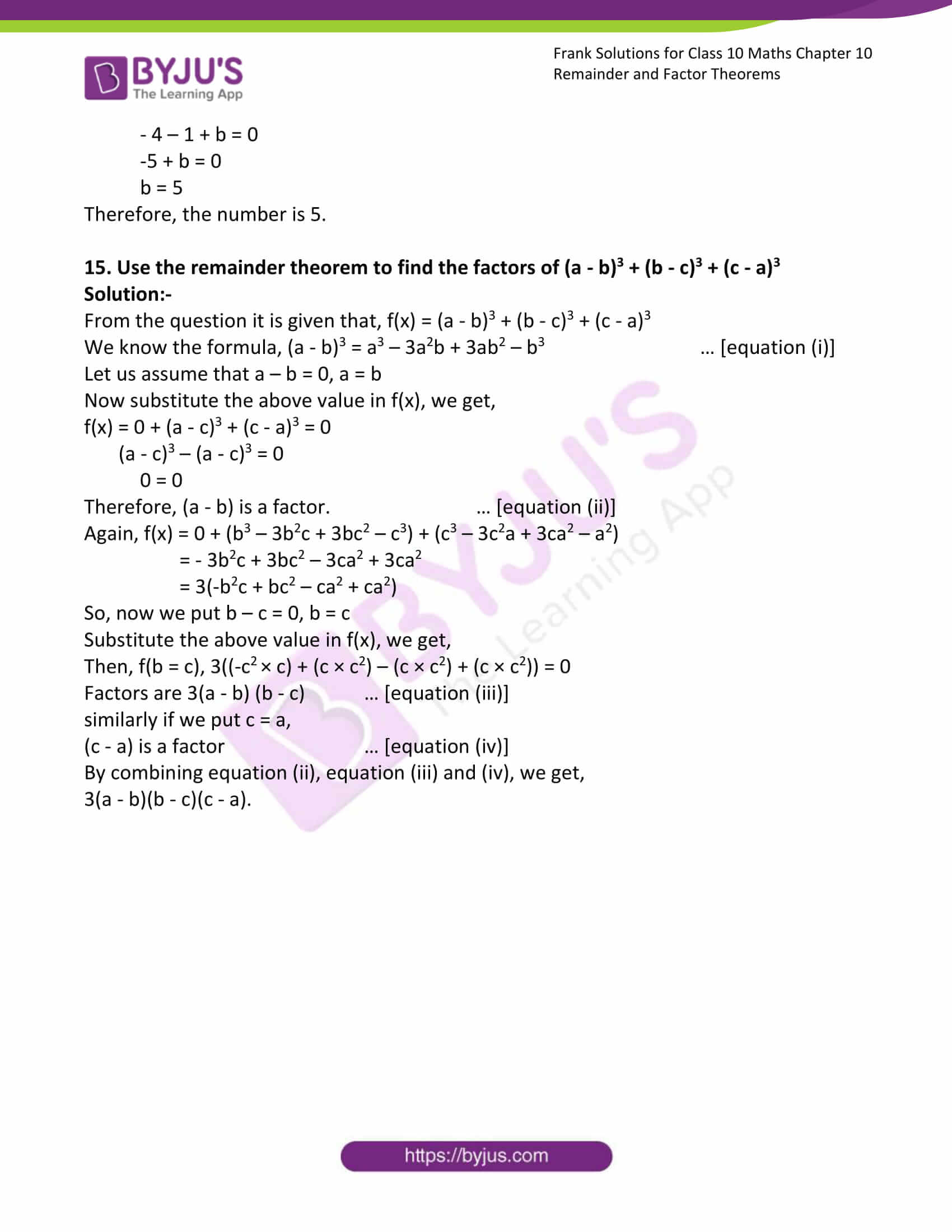### Access answers to Frank Solutions for Class 10 Maths Chapter 10 Remainder and Factor Theorems

1. Find without division, the remainder in each of the following:

(i) 5x2 – 9x + 4 is divided by (x – 2)

Solution:-

From the question it is given that, 5x2 – 9x + 4 is divided by (x – 2)

Let us assume x – 2 = 0, x = 2

Now, substitute the value of x in given expression,

= 5 × (2)2 – (9 × 2) + 4

= (5 × 4) – 18 + 4

= 20 – 18 + 4

= 24 – 18

= 6

Therefore, the remainder of the given expression is 6.

(ii) 5x3 – 7x2 + 3 is divided by (x – 1)

Solution:-

From the question it is given that, 5x3 – 7x2 + 3 is divided by (x – 1)

Let us assume x – 1 = 0, x = 1

Now, substitute the value of x in given expression,

= 5 × (1)2 – 7 × (1)2 + 3

= (5 × 1) – (7 × 1) + 3

= 5 – 7 + 3

= 8 – 7

= 1

Therefore, the remainder of the given expression 1.

(iii) 8x2 – 2x + 1 is divided by (2x + 1)

Solution:-

From the question it is given that, 8x2 – 2x + 1 is divided by (2x + 1)

Let us assume 2x + 1 = 0, x = -½

Now, substitute the value of x in given expression,

= 8(-½)2 – 2(-½) + 1

= (8 × 1/4) + 1 + 1

= 2 + 1 + 1

= 4

Therefore, the remainder of the given expression 4.

(iv) x3 + 8x2 + 7x – 11 is divided by (x + 4)

Solution:-

From the question it is given that, x3 + 8x2 + 7x – 11 is divided by (x + 4)

Let us assume x + 4 = 0, x = -4

Now, substitute the value of x in given expression,

= (-4)3 + 8(-4)2 + 7(-4) – 11

= – 64 + 8(-16) – 28 – 11

= -64 + 128 – 28 – 11

= 25

Therefore, the remainder of the given expression 25.

(v) 2x3 – 3x2 + 6x – 4 is divided by (2x – 3)

Solution:-

From the question it is given that, 2x3 – 3x2 + 6x – 4 is divided by (x + 4)

Let us assume 2x – 3 = 0, x = 3/2

Now, substitute the value of x in given expression,

= 2(3/2)3 – 3(3/2)2 + 6(3/2) – 4

= (2 × 27/8) – 3(9/4) + (3 × 3) – 4

= 27/4 – 27/4 + 9 – 4

= 9 – 4

= 5

Therefore, the remainder of the given expression 5.

2. Prove by factor theorem that,

(i) (x – 2) is a factor of 2x3 – 7x – 2

Solution:-

From the question it is given that, f(x) = 2x3 – 7x – 2

Let us assume, x – 2 = 0, x = 2

Then, substitute the value of x,

f(2) = 2(2)3 – 7(2) – 2

= 2(8) – 14 – 2

= 16 – 14 – 2

= 16 – 16

= 0

Now it is clear that (x – 2) is a factor of 2x3 – 7x – 2.

(ii) (2x + 1) is a factor of 4x3 + 12x2 + 7x + 1

Solution:-

From the question it is given that, f(x) = 4x3 + 12x2 + 7x + 1

Let us assume, 2x + 1 = 0, x = -½

Then, substitute the value of x,

f(-½) = 4 (-½)3 + 12 (-½)2 + 7 (-½) + 1

= 4(-1/8) + 12(1/4) – 7/2 + 1

= – ½ + 3 – 7/2 + 1

= – ½ – 7/2 + 4

= -8/2 + 4

= – 4 + 4

= 0

Now it is clear that (2x + 1) is a factor of 4x3 + 12x2 + 7x + 1.

(iii) (3x – 2) is a factor of 18x3 – 3x2 + 6x – 8

Solution:-

From the question it is given that, f(x) = 18x3 – 3x2 + 6x – 8

Let us assume, 3x – 2 = 0, x = 2/3

Then, substitute the value of x,

f(2/3) = 18 (2/3)3 – 3 (2/3)2 + 6 (2/3) – 8

= 18(8/27) – 3(4/9) + (2 × 2) – 8

= 2(8/3) – (4/3) + 4 – 8

= 16/3 – 4/3 + 4 – 8

= 12/3 + 4 – 8

= 4 + 4 – 8

= 8 – 8

= 0

Now it is clear that (3x – 2) is a factor of 18x3 – 3x2 + 6x – 8.

(iv) (2x – 1) is a factor of 6x3 – x2 – 5x + 2

Solution:-

From the question it is given that, f(x) = 6x3 – x2 – 5x + 2

Let us assume, 2x – 2 = 0, x = ½

Then, substitute the value of x,

f(½) = 6 (½)3 – (½)2 – 5 (½) + 2

= 6(1/8) – (1/4) – (5/2) + 2

= 3(1/4) – (1/4) – 5/2 + 2

= 3/4 – 1/4 – 5/2 + 2

= 2/4 – 5/2 + 2

= 1/2 – 5/2 + 2

= -4/2 + 2

= -2 + 2

= 0

Now it is clear that (2x – 1) is a factor of 6x3 – x2 – 5x + 2.

(v) (x – 3) is a factor of 5x2 – 21x + 18

Solution:-

From the question it is given that, f(x) = 5x2 – 21x + 18

Let us assume, x – 3 = 0, x = 3

Then, substitute the value of x,

f(3) = 5(3)2 – 21(3) + 18

= 5(9) – 63 + 18

= 45 – 63 + 18

= 63 – 63

= 0

Now it is clear that (x – 3) is a factor of 5x2 – 21x + 18.

3. Find the values of a and b in the polynomial f(x) = 2x3 + ax2 + bx + 10, if it is exactly divisible by (x + 2) and (2x – 1)

Solution:-

From the question it is given that,

f(x) = 2x3 + ax2 + bx + 10

let us assume x + 2 = 0

x = -2

2x – 1 = 0

x = ½

Now, substitute the value of x,

x = -2

f(-2) = 2(-2)3 + a(-2)2 + b(-2) + 10 = 0

2(-8) + a(4) – 2b + 10 = 0

– 16 + 4a – 2b + 10 = 0

– 6 + 4a – 2b = 0

Divide both side by 2 we get,

-6/2 + 4a/2 – 2b/2 = 0

– 3 + 2a – b = 0

2a = b + 3

a = b/2 + 3/2 … [equation (i)]

Then,

f(½) = 2(½)3 + a(½)2 + b(½) + 10 = 0

2(1/8) + a(1/4) + b/2 + 10 = 0

¼ + a/4 + b/2 + 10 = 0

Multiply by 4 for each terms we get,

1 + a + 2b + 40 = 0

41 + a + 2b = 0

a = – 2b – 41 … [equation (ii)]

By combining both equation (i) and equation (ii) we get,

b/2 + 3/2 = -2b – 41

(b + 3)/2 = -2b – 41

b + 3 = – 4b – 82

By transposing we get,

4b + b = -82 – 3

5b = – 85

b = -85/5

b = – 17

So, a = – 2b – 41

= -2(-17) – 41

= 34 – 41

= – 7

Therefore, the value of a is – 7 and b is – 17.

4. Using remainder theorem, find the value of m if the polynomial f(x) = x3 + 5x2 – mx + 6 leaves a remainder 2m when divided by (x – 1).

Solution:-

From the question it is given that,

f(x) = x3 + 5x2 – mx + 6

Remainder = 2m

Let us assume that x – 1 = 0, x = 1

Now, substitute the value of x in f(x) we get,

f(1) = 13 + 5(1)2 – m(1) + 6 = 2m

1 + 5 – m + 6 = 2m

6 – m + 6 = 2m

By transposing we get,

12 = 2m + m

12 = 3m

m = 12/3

m = 4

Therefore, the value of m is 4.

5. Find the value of m when x3 + 3x2 – mx + 4 is exactly divisible by (x – 2)

Solution:-

From the question it is given that,

f(x) = x3 + 3x2 – mx + 4

Let us assume that x – 2 = 0, x = 2

Now, substitute the value of x in f(x) we get,

f(2) = 23 + 3(2)2 – m(2) + 4 = 0

8 + 3(4) – 2m + 4 = 0

8 + 12 – 2m + 4 = 0

24 – 2m = 0

By transposing we get,

24 = 2m

m = 24/2

m = 12

Therefore, the value of m is 12.

6. Find the values of p and q in the polynomial f(x) = x3 – px2 + 14x – q, if it is exactly divisible by (x – 1) and (x – 2).

Solution:-

From the question it is given that, f(x) = x3 – px2 + 14x – q

Let us assume that, x – 1= 0, x = 1

x – 2 = 0, x = 2

Now, substitute the value of x in f(x) we get,

f(1) = 13 – p(1)2 + 14(1) – q = 0

1 – p + 14 – q = 0

15 – p – q = 0

p = 15 – q … [equation (i)]

Then, f(2) = 23 – p(2)2 + 14(2) – q = 0

8 – 4p + 28 – q = 0

36 – 4p – q = 0

q = 36 – 4p … [equation (ii)]

So, substitute the value of q in equation (i) we get,

p = 15 – (36 – 4p)

p = 15 – 36 + 4p

By transposing we get,

36 – 15 = 4p – p

21 = 3p

p = 21/3

p = 7

Consider the equation (ii) to find the value of q,

q = 36 – 4(7)

q = 36 – 28

q = 8

Therefore, the value of p is 7 and q is 8.

7. Find the values of a and b when the polynomial f(x) = ax3 + 3x2 + bx – 3 is exactly divisible by (2x + 3) and leaves a remainder – 3 when divided by (x + 2).

Solution:-

From the question it is given that, f(x) = ax3 + 3x2 + bx – 3

Remainder = -3

Let us assume that, 2x + 3 = 0, x = -3/2

x + 2 = 0, x = -2

Now, substitute the value of x in f(x) we get,

f(-3/2) = a(-3/2)3 + 3(-3/2)2 + b(-3/2) – 3= 0

a(-27/8) + 3(9/4) – b(3/2) – 3 = 0

a(-27/8) + (27/4) – 3 – b(3/2) = 0

a(-27/8) + (27- 12)/4 – b(3/2) = 0

a(-27/8) + 15/4 – b(3/2) = 0

-27a + 30 – 12b = 0

27a = – 12b + 30

Then,

f(- 2) = a(-2)3 + 3(-2)2 + b(-2) – 3= -3

a(-8) + 3(4) – 2b – 3 = -3

-8a + 12 – 2b – 3 + 3= 0

-4a + 6 – b = 0

b = 6 – 4a … [equation (ii)]

By combining both equation (i) and equation (ii) we get,

27a = – 12(6 – 4a) + 30

27a = – 72 + 48a + 30

27a – 48a = – 42

– 21a = -42

a = -42/-21

a = 2

Then,

b = 6 – 4a

b = 6 – 4(2)

b = 6 – 8

b = -2

Therefore, the value of a is 2 and b is – 2.

8. Find the values of m and n when the polynomial f(x) = x3 – 2x2 + mx + n has a factor (x + 2) and leaves a remainder 9 when divided by (x + 1).

Solution:-

From the question it is given that,

f(x) = x3 – 2x2 + mx + n

Remainder = 9

Let us assume that, x + 2 = 0, x = – 2

x + 1 = 0, x = -1

Now, substitute the value of x in f(x) we get,

f(-2) = (-2)3 – 2(-2)2 + m(-2) + n = 0

– 8 – 8 – 2m + n = 0

– 16 – 2m + n = 0

n = 2m + 16 … [equation (i)]

Then, f(-1) = (-1)3 – 2(-1)2 + m(-1) + n = 9

– 1 – 2 – m + n = 9

– 3 – m + n = 9

m = – 3 – 9 + n

m = n – 12 … [equation (ii)]

Now, combining both equation (i) and equation (ii) we get,

n = 2(n – 12) + 16

n = 2n – 24 + 16

n = 2n – 8

2n – n = 8

n = 8

Consider the equation (ii) to find out the value of m,

m = n – 12

m = 8 – 12

m = -4

Therefore, the value of n is 8 and m is – 4.

9. Find the values of a and b when the polynomials f(x) = 2x2 – 5x + a and g(x) = 2x2 + 5x + b both have a factor (2x + 1)

Solution:-

From the question it is given that,

f(x) = 2x2 – 5x + a

g(x) = 2x2 + 5x + b

Let us assume 2x + 1 = 0, x = -½

Now, substitute the value of x in f(x) we get,

f(-½) = 2(-½)2 – 5(-½) + a = 0

2(1/4) + 5/2 + a = 0

½ + 5/2 + a = 0

6/2 + a = 0

3 + a = 0

a = – 3

Then,

g(-½) = 2(-½)2 + 5(-½) + b = 0

2(1/4) – 5/2 + b = 0

½ – 5/2 + b = 0

– 4/2 + b = 0

– 2 + b = 0

b = 2

Therefore, the value of a is – 3 and b is 2.

10. Find the values of a and b when the factors of the polynomial f(x) = ax3 + bx2 + x – a are (x + 3) and (2x – 1). Factorize the polynomial completely.

Solution:-

From the question it is given that, f(x) = ax3 + bx2 + x – a

Let us assume, x + 3 = 0, x = -3

2x – 1 = 0, x = ½

Now, substitute the value of x in f(x) we get,

f(-3) = a(-3)3 + b(-3)2 + (-3) – a = 0

-27a + 9b – 3 – a = 0

-28a + 9b – 3 = 0

By transposing we get,

-28a = -9b + 3

a = -9b/-28 + 3/-28

a = 9b/28 – 3/28 … [equation (i)]

Then, f(½) = a(½)3 + b(½)2 + (½) – a = 0

a(1/8) + b(1/4) + ½ – a= 0

(a – 8a)/8 + b(1/4) + ½ = 0

-7a/8 + b(¼) + ½ = 0

b(¼) = -½ + 7a/8

b = -4/2 + 28a/8

b = -2 + 7a/2 … [equation (ii)]

Now, combining equation (i) and equation (ii) we get,

a = 9/28 × (7a/2 – 2) – 3/28

By simplification we get,

56a = 63a – 42

a = 6

Consider the equation (ii) to find out the value of b,

b = 7a/2 – 2

b = (7 × 6)/2 – 2

b = 42/2 – 2

b = 21 – 2

b = 19

Substitute the value of a and b in f(x) we get,

f(x) = 6x3 + 19x2 + x – 6

Therefore, equation becomes (x + 3) (2x – 1) (3x + 2) = 0

11. What number should be subtracted from x2 + x + 1 so that the resulting polynomial is exactly divisible by (x – 2)?

Solution:-

From the question it is given that, f(x) = x2 + x + 1

Then, x – 2 = 0, x = 2

Let us assume the number should be subtracted from x2 + x + 1 be b,

f(2) = 22 + 2 + 1 – b = 0

4 + 2 + 1 – b = 0

7 – b = 0

b = 7

Therefore, the number is 7.

12. What number should be added to 2x3 – 3x2 + 7x – 8 so that the resulting polynomial is exactly divisible by (x – 1)?

Solution:-

From the question it is given that, f(x) = 2x3 – 3x2 + 7x – 8

Then, x – 1 = 0, x = 1

Let us assume the number should be added to 2x3 – 3x2 + 7x – 8 be b,

f(1) = 2(1)3 – 3(1)2 + 7(1) – 8 + b = 0

2 – 3 + 7 – 8 + b = 0

9 – 11 + b = 0

– 2 + b = 0

b = 2

Therefore, the number is 2.

13. What number should be subtracted from polynomial f(x) 2x3 – 5x2 + 8x – 17 so that the resulting polynomial is exactly divisible by (2x – 5)?

Solution:-

From the question it is given that, f(x) = 2x3 – 5x2 + 8x – 17

Then, 2x – 5 = 0, x = 5/2

Let us assume the number should be subtracted from 2x3 – 5x2 + 8x – 17 be b,

f(5/2) = 2(5/2)3 – 5(5/2)2 + 8(5/2) – 17 – b = 0

2(125/8) – 5(25/4) + 40/2 – 17 – b = 0

125/4 – 125/4 + 20 – 17 – b = 0

3 – b = 0

b = 3

Therefore, the number is 3.

14. What number should be added to polynomial f(x) 12x3 + 16x2 – 5x – 8 so that the resulting polynomial is exactly divisible by (2x – 1)?

Solution:-

From the question it is given that, f(x) = 12x3 + 16x2 – 5x – 8

Then, 2x – 1 = 0, x = ½

Let us assume the number should be added to 2x3 – 3x2 + 7x – 8 be b,

f(½) = 12(½)3 + 16(½)2 – 5(½) – 8 + b = 0

12(1/8) + 16(1/4) – 5/2 – 8 + b = 0

3/2 + 4 – 5/2 – 8 + b = 0

– 4 – 2/2 + b = 0

– 4 – 1 + b = 0

-5 + b = 0

b = 5

Therefore, the number is 5.

15. Use the remainder theorem to find the factors of (a – b)3 + (b – c)3 + (c – a)3

Solution:-

From the question it is given that, f(x) = (a – b)3 + (b – c)3 + (c – a)3

We know the formula, (a – b)3 = a3 – 3a2b + 3ab2 – b3 … [equation (i)]

Let us assume that a – b = 0, a = b

Now substitute the above value in f(x), we get,

f(x) = 0 + (a – c)3 + (c – a)3 = 0

(a – c)3 – (a – c)3 = 0

0 = 0

Therefore, (a – b) is a factor. … [equation (ii)]

Again, f(x) = 0 + (b3 – 3b2c + 3bc2 – c3) + (c3 – 3c2a + 3ca2 – a2)

= – 3b2c + 3bc2 – 3ca2 + 3ca2

= 3(-b2c + bc2 – ca2 + ca2)

So, now we put b – c = 0, b = c

Substitute the above value in f(x), we get,

Then, f(b = c), 3((-c2 × c) + (c × c2) – (c × c2) + (c × c2)) = 0

Factors are 3(a – b) (b – c) … [equation (iii)]
similarly if we put c = a,

(c – a) is a factor … [equation (iv)]

By combining equation (ii), equation (iii) and (iv), we get,

3(a – b)(b – c)(c – a).